# Circular Motion Questions and Answers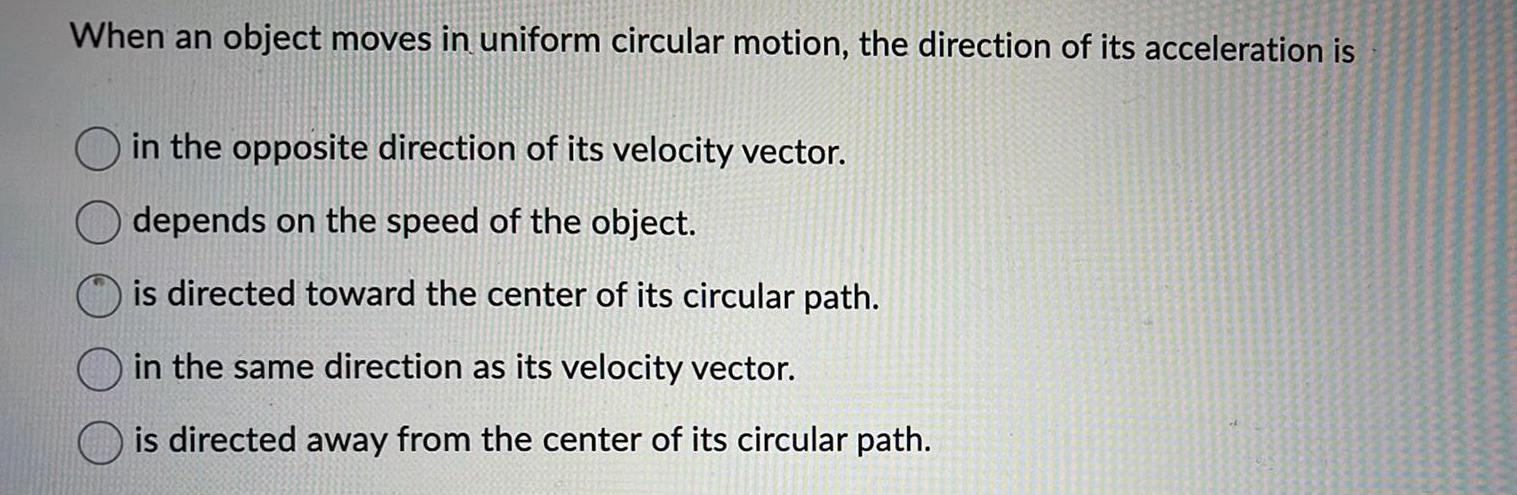Physics
Circular Motion
When an object moves in uniform circular motion, the direction of its acceleration is in the opposite direction of its velocity vector. depends on the speed of the object. is directed toward the center of its circular path. in the same direction as its velocity vector. is directed away from the center of its circular path.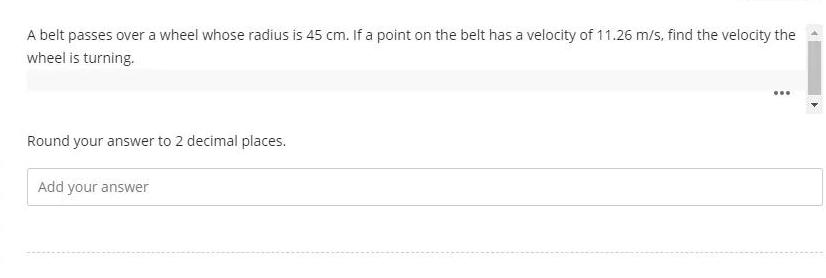Physics
Circular Motion
A belt passes over a wheel whose radius is 45 cm. If a point on the belt has a velocity of 11.26 m/s, find the velocity the wheel is turning. Round your answer to 2 decimal places.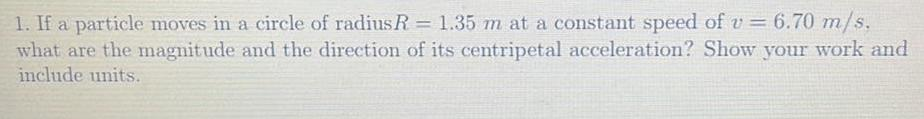Physics
Circular Motion
1. If a particle moves in a circle of radius R = 1.35 m at a constant speed of v= 6.70 m/s. what are the magnitude and the direction of its centripetal acceleration? Show your work and include units.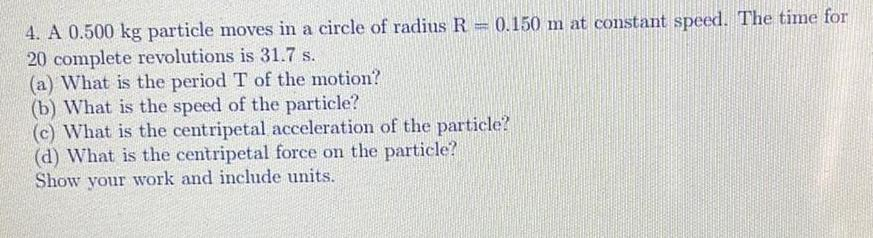Physics
Circular Motion
4. A 0.500 kg particle moves in a circle of radius R = 0.150 m at constant speed. The time for 20 complete revolutions is 31.7 s. (a) What is the period T of the motion? (b) What is the speed of the particle? (c) What is the centripetal acceleration of the particle? (d) What is the centripetal force on the particle? Show your work and include units.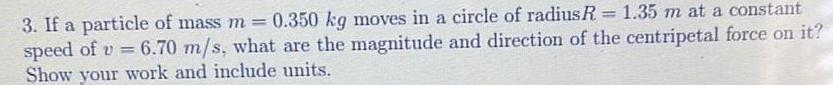Physics
Circular Motion
3. If a particle of mass m=0.350 kg moves in a circle of radius R = 1.35 m at a constant speed of v= 6.70 m/s, what are the magnitude and direction of the centripetal force on it? Show your work and include units.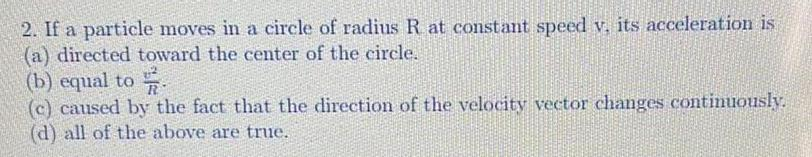Physics
Circular Motion
If a particle moves in a circle of radius R at constant speed v, its acceleration is (a) directed toward the center of the circle. (b) equal to v2/R (c) caused by the fact that the direction of the velocity vector changes continuously. (d) all of the above are true.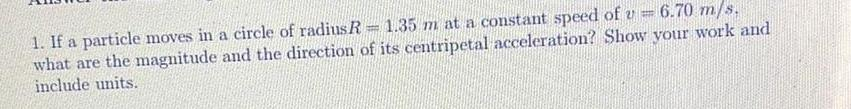Physics
Circular Motion
1. If a particle moves in a circle of radius R = 1.35 m at a constant speed of u = what are the magnitude and the direction of its centripetal acceleration? Show your work and include units.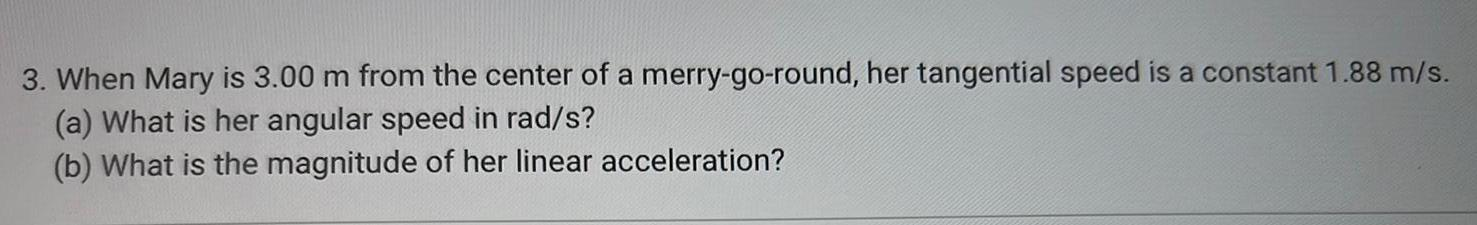Physics
Circular Motion
When Mary is 3.00 m from the center of a merry-go-round, her tangential speed is a constant 1.88 m/s. (a) What is her angular speed in rad/s? (b) What is the magnitude of her linear acceleration?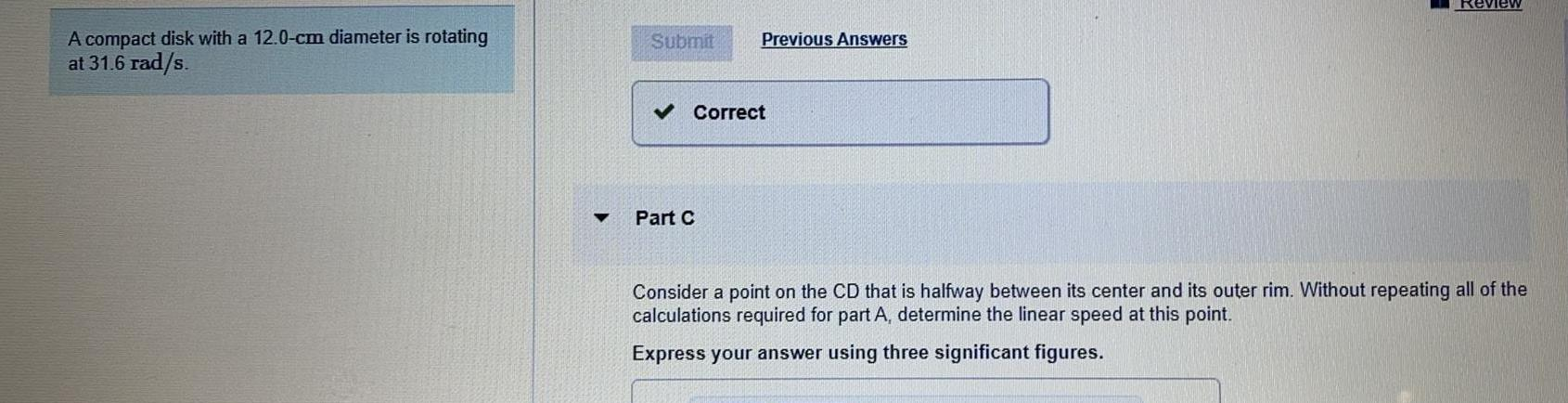Physics
Circular Motion
A compact disk with a 12.0-cm diameter is rotating at 31.6 rad/s. Part C Consider a point on the CD that is halfway between its center and its outer rim. Without repeating all of the calculations required for part A, determine the linear speed at this point. Express your answer using three significant figures.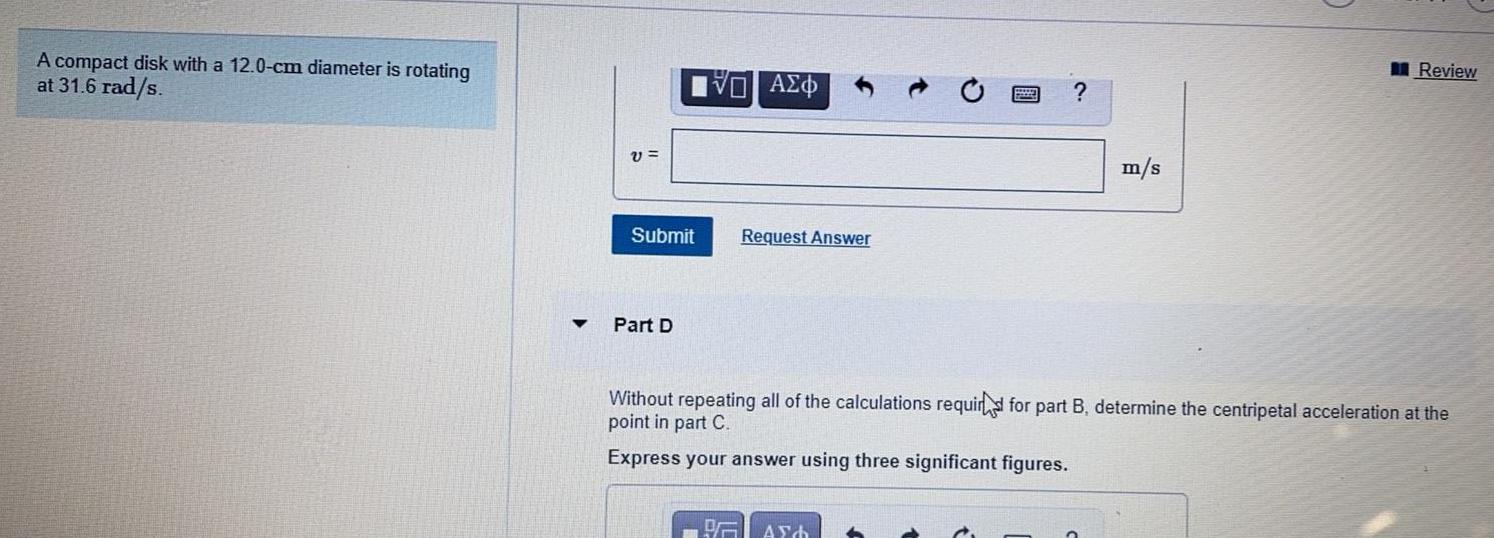Physics
Circular Motion
A compact disk with a 12.0-cm diameter is rotating at 31.6 rad/s. Without repeating all of the calculations requird for part B, determine the centripetal acceleration at the point in part C.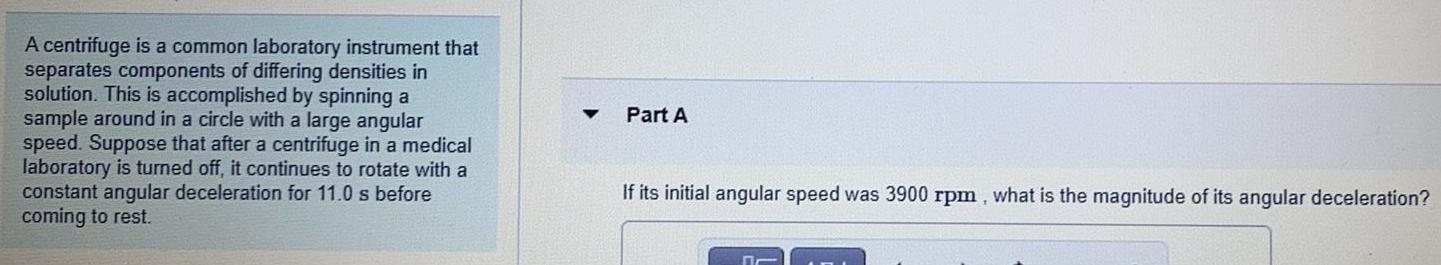Physics
Circular Motion
A centrifuge is a common laboratory instrument that separates components of differing densities in solution. This is accomplished by spinning a sample around in a circle with a large angular speed. Suppose that after a centrifuge in a medical laboratory is turned off, it continues to rotate with a constant angular deceleration for 11.0 s before coming to rest. If its initial angular speed was 3900 rpm, what is the magnitude of its angular deceleration?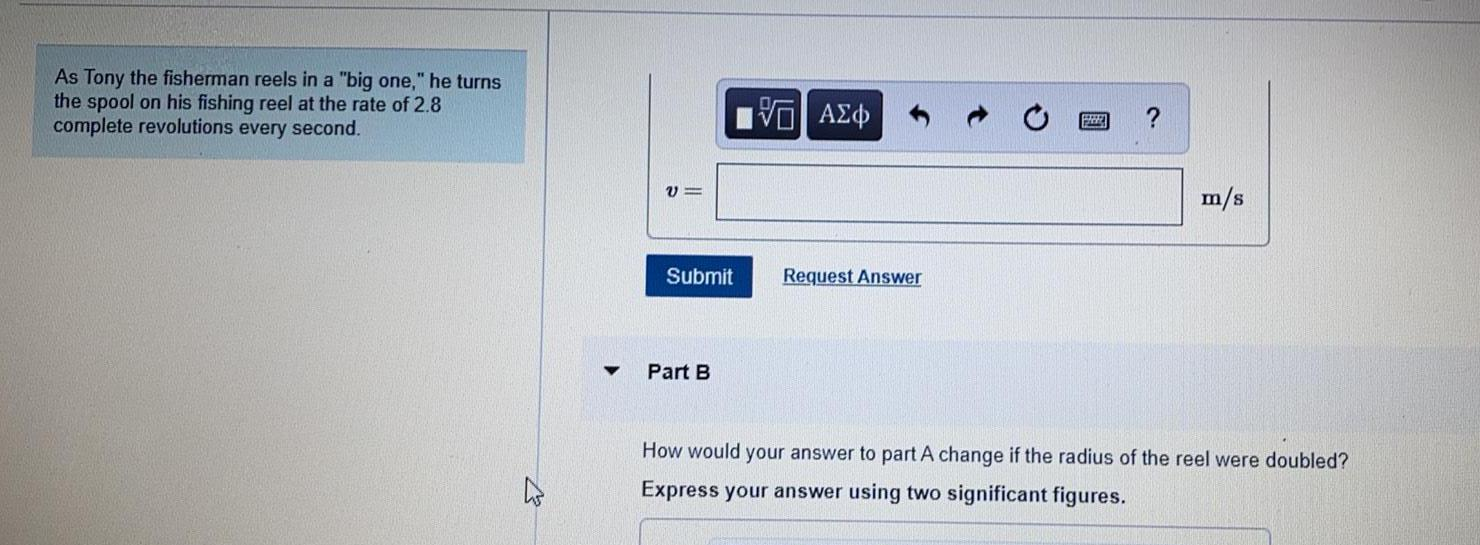Physics
Circular Motion
As Tony the fisherman reels in a "big one," he turns the spool on his fishing reel at the rate of 2.8 complete revolutions every second. How would your answer to part A change if the radius of the reel were doubled? Express your answer using two significant figures.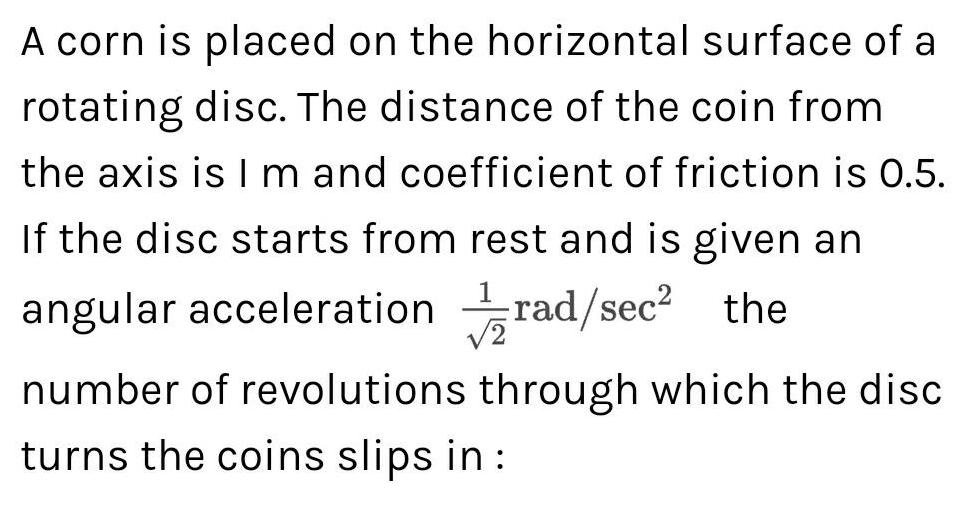Physics
Circular Motion
A corn is placed on the horizontal surface of a rotating disc. The distance of the coin from the axis is I m and coefficient of friction is 0.5. If the disc starts from rest and is given an angular acceleration 1/√2 rad/sec² the number of revolutions through which the disc turns the coins slips in: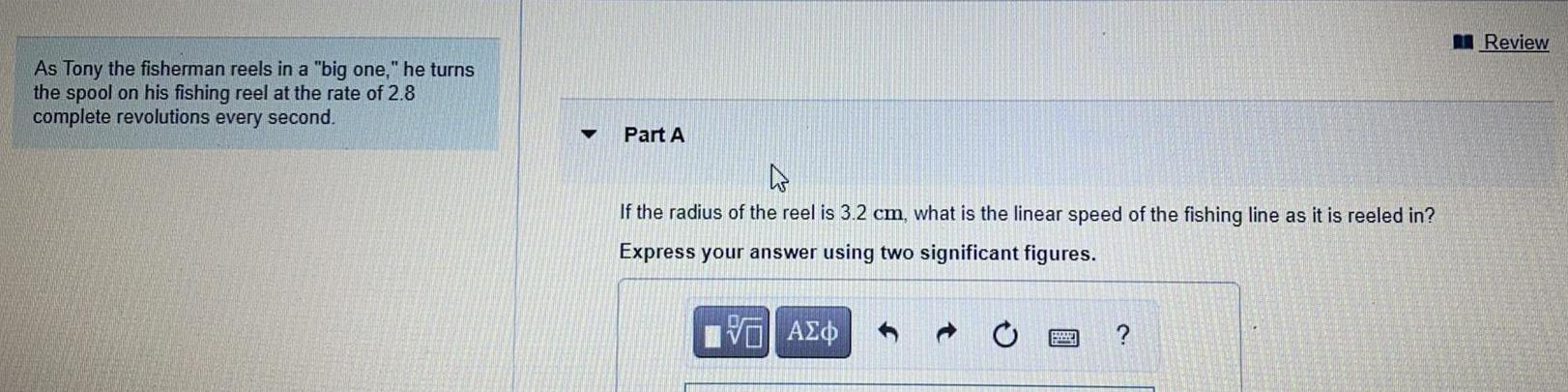Physics
Circular Motion
As Tony the fisherman reels in a "big one," he turns the spool on his fishing reel at the rate of 2.8 complete revolutions every second. ▼ Part A If the radius of the reel is 3.2 cm, what is the linear speed of the fishing line as it is reeled in? Express your answer using two significant figures.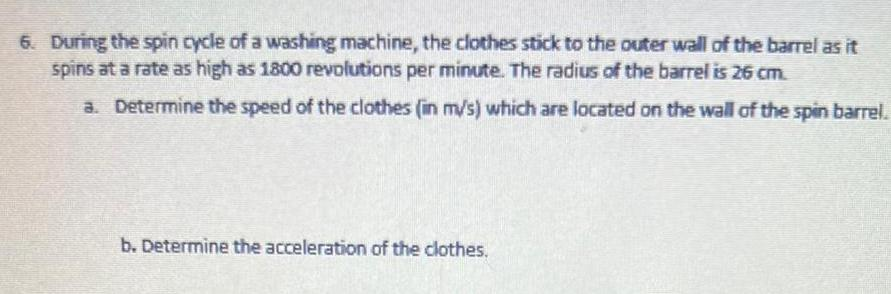Physics
Circular Motion
6. During the spin cycle of a washing machine, the clothes stick to the outer wall of the barrel as it spins at a rate as high as 1800 revolutions per minute. The radius of the barrel is 26 cm. a. Determine the speed of the clothes (in m/s) which are located on the wall of the spin barrel. b. Determine the acceleration of the clothes.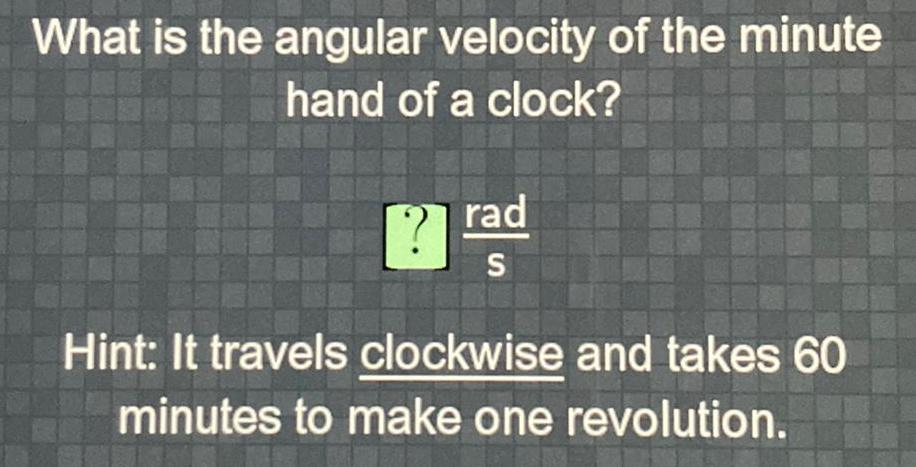Physics
Circular Motion
What is the angular velocity of the minute hand of a clock? Hint: It travels clockwise and takes 60 minutes to make one revolution.